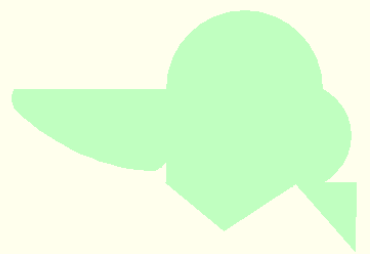Spike's Calculators

# Area Calculator with Multiple ShapesThis calculator will let you select shapes as needed and can be useful for odd shape area calculation. Included is a field for depth which will calculate the volume for an area, in case you are looking to add something that would require a volume measurement.

Added an example of an odd shaped area which looks like a head of some one with a very large beak.

Of course this example has been designed with the calculator in mind, but it should give you the general idea how to calculate area of a funny looking hard to calculate form.

This area can be split up in to various segments that can be calculated. Look at the shapes that come with the calculator to see for which measurements area required for each shape.rectangle: 1' x 24'
half circle: 41'
circle segment: 24' x 7' 6"
right angled triangle: 18' x 15'
right angled triangle: 17' 6" x 30' 6"
scalene triangle: 33'6" x 19' 6" x 22'As you will notice the hypotenuse of a right angled triangle is not needed. The hypotenuse will get calculated and in this case it can be used for the major axis of the half oval. How lucky can you get!!

Calculate this triangle first to get the measurement needed for the half oval.
Right angled triangle result: 17' 6" x 30' 6"
hypotenuse = 35.2'
area = 266.9 square feet
perimeter = 83.2'
volume = 66.7 cubic feet

The results are in decimal format which is also the format that the measurements need to be entered in 30' 6" in decimal format = 30.5 and from decimal format 83.2 becomes 83' 2 3/8" with a wee bit of rounding. But in the scope of things I doubt you need to be that precise.

The results for each shape are not identical, but includes missing lengths such as arc, hypotenuse and the leg size of a trapezoid.

It will keep track of total square footage calculated for area and if a value for depth is used , the total volume in cubic feet. For instance if you entered a value for depth it would give you a total volume in the end that could be used for topsoil needed or if you wanted to pour a concrete slab, the cubic feet of concrete needed.

For interest sake the total estimated area of the above image shape = 2482 square feet and if you were to pour a 3" slab on this you would need 591 cubic feet of concrete.

### The total results will appear here:

 Total Area: Total Volume: #